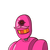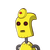# Subtract 3x – 4y – 7z from the sum of x – 3y + 2z and -4x + 9y – 11z​

Subtract 3x – 4y – 7z from the sum of x – 3y + 2z and -4x + 9y – 11z​

### 2 thoughts on “Subtract 3x – 4y – 7z from the sum of x – 3y + 2z and -4x + 9y – 11z​”

1.according to the question,

• x – 3y + 2z + (-4x + 9y – 11z)
• -3x + 6y -9z

now,

• -3x + 6y -9z – (3x – 4y -7z)
• -3x + 6y -9z -3x + 4y + 7z
• -6x + 10y – 2z

2.= x – 3y + 2z + (-4x) + 9y – 11z – (3x – 4y – 7z)

= x – 3y + 2z – 4x + 9y – 11z – 3x + 4y + 7z

= x – 4x – 3x + 2z – 11z + 7z + 9y + 4y

= -3x – 3x + 2z – 4z + 13y

= -6x – 2z + 13y

Step-by-step explanation:

Hope this helps you.

# By Sparkly Princess